# GSEB Solutions Class 9 Maths Chapter 8 Quadrilaterals Ex 8.1

Gujarat Board GSEB Solutions Class 9 Maths Chapter 8 Quadrilaterals Ex 8.1 Textbook Questions and Answers.

## Gujarat Board Textbook Solutions Class 9 Maths Chapter 8 Quadrilaterals Ex 8.1

Question 1.
The angles of a quadrilateral are in the ratio 3 : 5 : 9 : 13. Find all the angles of the quadrilateral.
Solution:
We have
∠A : n∠B : ∠C : ∠D = 3 : 5 : 9 : 13
Let ∠A = 3x,
∠B = 5x,
∠C = 9x,
and ∠D = 13x
∠A + ∠B + ∠C + ∠D
Sum of all angles of a quadrilateral
3x + 5x + 9x + 13x = 360°
⇒ 30 x = 360°
x = $$\frac {360°}{30}$$
⇒ x = 12°
Hence, ∠A = 3x = 3 x 12° = 36°
∠B = 5x = 5 x 12° = 60°
∠C = 9x = 9 x 12° = 108°
and ∠D = 13 = 13 x 12° = 156°Question 2.
If the diagonals of a parallelogram are equal, then show that it is a rectangle.
Solution:
Given: ABCD is a parallelogram in which AC = BD.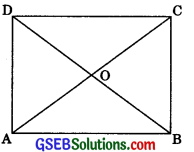To prove: ABCD is a rectangle.
AB = BA (Common)
BC – AD (Opposite sides of a parallelogram)
AC = BD (Given)
∴ ΔABC s ΔBAD (by SSS congruency)
Hence ∠ABC = ∠BAD (by CPCT)
∠ABC + ∠BAD = 180° (Consecutive interior
angles)
⇒ 2∠BAD = $$\frac {180°}{2}$$
Therefore, ABCD is a rectangle.

Question 3.
Show that if the diagonals of a quadrilateral bisect each other at right angles, then it is a rhombus.
Solution:
Given: ABCD is a quadrilateral in which diagonals AC and BD bisect each other at right angle, i.e., ∠AOB = 90°.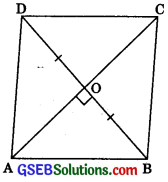To Prove: ABCD is a rhombus.
Proof: In ΔAOB and ΔAOD,
OB = OD (Given)
∠AOB = ∠AOD (each 90°)
OA = OA (Common)
∴ ΔAOB ≅ ΔAOD (by SAS congruency)
Hence, AB = AD …….(1) (by CPCT)
Similarly, we can prove
AB = BC ……(2)
and BC = CD …….(3)
AB = BC = CD = AD [From (1), (2) and (3)]
Hence, ABCD is a rhombus.Question 4.
Show that the diagonals of a square are equal and bisect each other at right angles.
Solution:
Given: ABCD is a square in which AC and BD are diagonals.
To Prove: (i) AC = BD
(ii) AC and BD bisect each other at right angles.Proof: (i) In ΔABC and ΔBAD,
AB = BA (Common)
BC = AD (Opposite sides of a square)
ΔABC – ΔBAD (by SAS congruency)
Therefore AC = BD (by CPCT)

(iii) Now in ΔAOB and ΔCOD,
∠AOB = ∠COD (Vertically opposite angles)
∠OAB = ∠OCD (Alternate interior angles)
AB = CD (Opposite side of a square)
∴ ΔAOB – ΔCOD (by AAS congruency)
Hence, OA = OC (by CPCT)
OB = OD (by CPCT)
Now in ΔAOB and ΔBOC,
OA = OC (proved above)
AB = BC (Side of a square)
∴ ΔAOB = ΔBOC (by SSS congruency)
∴ ∠AOB = ∠BOC (by CPCT)
∠AOB + ∠BOC = 180° (Linear pair axiom)
⇒ ∠BOC + ∠BOC = 180°
⇒ 2∠BOC = 180°
⇒∠BOC = $$\frac {180°}{2}$$
∴ ∠BOC = 90°
Hence AC and BD bisect each other at right angles.Question 5.
Show that if the diagonals of a quadrilateral are equal and bisect each other at right angles, then it is a square.
Solution:
Given: In Quadrilateral ABCD, AC = BD and OA = OC, OB = OD and ∠AOB = 90°.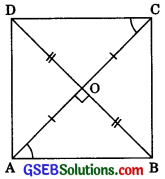To Prove: ABCD is a square.
Proof In ΔAOB and ΔCOÐ
OA = OC (given)
∠AOB = ∠COD (each 90°)
OB = OD (given)
ΔAOB-ΔCOD (by SAS congruency)
AB = CD (by CPCT)
∠OAB = ∠OCD (by CPCT)
(Alternate interior angles)
⇒ AB || CD
Hence ABCD is a parallelogram.
Now in ΔAOB and ΔBOC
OA = OC (given)
∠AOB = ∠BOC (each 90°)
OB = OB (common)
∴ ΔAOB – ΔBOC
(by SAS congruency)
Hence AB = BC (by CPCT)
Similarly, we can prove BC = CD
and CD = DA
AB = BA (common)
(Opposite sides of a parallelogram)
AC = BD (given)
∴ ΔABC = ΔBAD (by SSS congruency)
Hence ∠ABC = ∠BAD (by CPCT)
∠ABC + ∠BAD = 180° (Consecutive interior angles of ||gm)

Thus, ABCD is a square.Question 6.
Diagonal AC of a parallelogram ABCD bisects ∠A. Show that
(i) it bisects ∠C also,
(ii) ABCD is a rhombus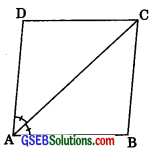Solution:
Given: ABCD is a parallelogram.
∠1 = ∠2
To Prove: ∠3 = ∠4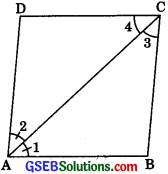Proof: (i) ∠1 = ∠2 …….(1) (given)
AB || CD
∴ ∠1 = ∠4 ……..(2)
(Alternate interior angles)
∴ ∠2 = ∠3 …….(3)
(Alternate interior angles)
From eqn. (1), (2) and (3)
∠3 = ∠4
Hence, AC bisects ∠C.
(ii) From eqn. (1) and (3), we get
∠1 = ∠3
∴ AB = BC
(Sides opposite to equal angles are equal)Similarly, from eqn. (1) and (2),
∠2 = ∠4
But AB = DC
Hence AB = BC = DC = AD
Therefore, ABCD is a rhombus.Question 7.
ABCD is a rhombus. Show that diagonal AC bisects ∠A as well as ∠C and diagonal BD bisects ∠B as well as ∠D.
Solution:
Given: Rhombus ABCD, in which AC and BD are diagonals.
To Prove:
(i) Diagonal AC bisects ∠A as well as ∠C.
(ii) Diagonal BD bisects ∠B as well as ∠D.
Proof: (i) ABCD is a rhombus.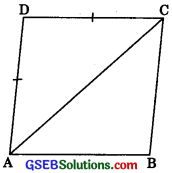AD = DC (Sides of rhombus)
∴ ∠DAC = ∠DCA ………(1)
(Angle opposite to equal sides are equal)
AB || DC (Opposite sides of rhombus)
∴ ∠BAC = ∠DCA …….(2)
(Alternate interior angles)
From eqn. (1) and (2),
∠BAC = ∠DAC
∴ Diagonal AC bisects ∠A.
Similarly, we can prove
∠BCA = ∠DCA
Hence diagonal AC also bisects ZC.

(ii) BC = DC (Sides of rhombus)
∠DBC = ∠BDC …….(3)
(Angles opposite to equal sides are equal)
AB || DC
(Opposite sides of rhombus)
∠ABD = ∠BDC …….(4)
(Alternate interior angles)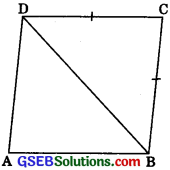From eqn. (3) and (4)
∠DBC = ∠ABD Hence diagonal BD bisects ∠B.
Similarly, we can prove diagonal BD bisects ∠D.Question 8.
ABCD is a rectangle in which diagonal AC bisects ∠A as well ∠C. Show that:
(i) ABCD is a square.
(ii) Diagonal BD bisects ∠B as well as ∠D.
Solution: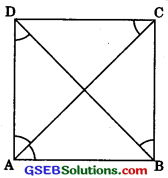AB || DC
(Opposite sides of a rectangle) ∠BAC = ∠DCA ……(1)
(Alternate interior angle) But ∠BAC = ∠DAC …….(2) (given)
From eqn. (1) and (2)
∠DCA = ∠DAC
(Sides opposite to equal angles are equal)
Hence ABCD is a square.

(Opposite sides of a square)
(Alternate interior angle)
But AB = AD (Side of square)
(Angle opposite sides to equal are equal)

From (3) and (4)
∠ABD = ∠CBD …….(4)
Hence diagonal BD bisects ZB as well ZD.

Question 9.
In parallelogram ABCD, two points P and Q are taken on diagonal BD such that DP = BQ. Show that
(i) AAPD = ACQB
(ii) AP = CQ
(iii) ΔAQB = ΔCPD
(iv) AQ = CP
(v) APCQ is a parallelogram.
Solution: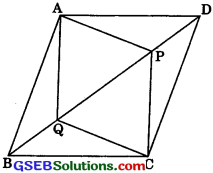To Prove:
(i) In ΔAPD and ΔCQB,
(Opposite sides of a parallelogram)
(Alternate interior angles)
PD = BQ (given)
∴ ΔAPD = ΔCQB
(by SAS congruency)

(ii) ΔAPD = ΔCQB (Proved above)
Hence AP = CQ …….(1) (by CPCT)(iii) In ΔAQB and ΔCPD,
AB = CD
(Opposite sides of a parallelogram)
AB|| CD
∴ ∠ABD = ∠CDB
(Alternate interior angles)
∠ABQ = ∠CDP
BQ = PD (given)
Hence, AAQB – ACPD
(by SAS congruency)

(iv) ΔABQ = ΔCPD (Proved above)
AQ = CP …….(2) (by CPCT)

(v) From eqn. (1) and (2)
APCQ is a prallelogram.
(If each pair of opposite sides of a quadrilat¬eral are equal then it is a parallelogram).

Question 10.
ABCD is a parallelogram and AP and CQ are perpendiculars from vertices A and C on diagonal BD respectively (See figure). Show that
(i) ΔAPB = ΔCQD
(ii) AP = CQ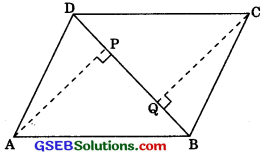Solution:
Given: ABCD is a parallelogram and AP ⊥ BD and CQ ⊥ BD respectively.
(i) In ΔAPB and ACQD,
AB = DC
(Opposite sides of a parallelogram)
AB || CD
∴ ∠ABP = ∠CDQ
(Alternate interior angles)
∠APB = ∠CQD (each 90°)
Hence, ΔAPB s ΔCQD
(by AAS congruency)

(ii) ΔAPB = ΔCQD (Proved above)
Hence, AP = CQ (by CPCT)Question 11.
In ΔABC and ΔDEF, AB = DE, AB || DE, BC = EF and BC || EF, vertices A, B and C are joined to verticals D, E and F respectively (see figure). Show that
(i) Quadrilateral ABED is a parallelogram.
(ii) Quadrilateral BEFC is a parallelogram.
(iv) Quadrilateral ACFD is a parallelogram.
(v) AC = DF
(vi) ΔABC = ΔDEF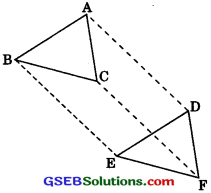Solution:
(i) In ΔABC and ΔDEF
AB = DE (given)
AB || DE (given)
∴ ABED is a parallelogram.
(In a quadrilateral if one pair of opposite sides is equal and parallel then it is a parallelogram).

(ii) In ΔABC and ΔDEF
BC = EF (given)
BC || EF (given)
∴ BEFC is a parallelogram.
(If one pair of opposite sides of a quadrilateral is equal and parallel then, it is a parallelogram).(iii) ABED is a parallelogram. (Proved above)
and BEFC is a parallelogram.
(Proved above)
BE =CF
BE || CF ……(2)
From eqn. (1) and (2)

(iv) AD || CF (Proved above)
∴ ACFD is a parallelogram.
(If one pair of opposite side of a quadrilateral is equal and parallel, then it is a parallelogram)

(v) ACFD is a parallelogram
∴ AC = DF
(Opposite sides of a parallelogram)

(vi) Now in ΔABC and ΔDEF,
BC = EF (given)
AB = DE (given)
and AC = DF (Proved above)
∴ ΔABC = ΔDEF (by SSS congruency).

Question 12.
ABCD is a trapezium in which AB || CD and AD = BC (see figure). Show that
(i) ∠A = ZB
(ii) ∠C = ZD
(iv) Diagonal AC = diagonal BD.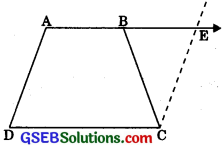Solution:
Given: ABCD is a trapezium in which AB || CD and AD = BC.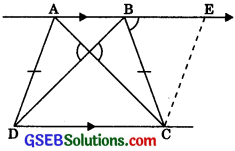Construction: Extend AB and draw a line through C parallel to DA intersecting AB produced at E.
Proof:
(i) AB || DC (Given)
AE || DC
and AD || CE (by construction)
(Both pair of opposite sides of a quadrilateral are parallel)
(Opposite angles of a parallelogram)
(Opposite sides of a parallelogram)
Hence BC = CE
∠CBE = ∠CEB
(Angle opposite to equal sides are equal)
∠CBE = ∠AEC ……(1) (∠AEC = ∠CEB) ∠DAB + ∠AEC = 180° …….(2)
(Consecutive interior angles)
∠ABC + ∠CBE = 180° …….(3) (Linear pair)From eqn. (1) and (3),
∠ABC = ∠AEC = 180° ……(4)
Now from eqn. (2) and (4),
∠DAB + ∠AEC = ∠ABC + ∠AEC
∠DAB = ∠ABC

(ii) AB || DC ∠DAB + ∠ADC = 180°
(Consecutive interior angles)
∠DAB = ∠ABC
(Proved above)
∠ABC + ∠ADC = 180° ……(5)
and ∠ABC + ∠BCD = 180° …….(6)
(Consecutive interior angles) From eqn. (5) and (6),
∠ABC + ∠ADC = ∠ABC + ∠BCD